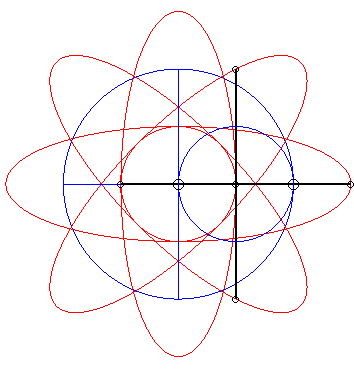Fascinating Spirographvia Reddit

Fascinating animation found on Reddit. The math boggles my tiny mind:

Point on the horizontal segment: x(t)

Point on the vertical segment: y(t)

x(t) = cos(a*t);
y(t) = sin(a*t);

a = 2 * pi * f (frequency of the 1 dimensional “wave” each part follows)

The stationary blue circle is generated by the vector sum of x(t) and y(t). In other words, it’s at a distance of d, where d(t) = sqrt(x(t)2 + y(t)2 ) (Pythagorean theorem), and at an angle of theta(t) = arctan(y(t)/x(t)) (trig) at any given time t.

The center red circle is given by the vector sum of x(t)/2 and y(t)/2.

The vertical and horizontal ellipses (ovals) are simple to see, but harder to understand the math behind them from just the animation.

For the vertical red oval, its y coordinate is always double the original y(t). Its x coordinate is always 1/2 x(t). Lets call this oval 1.

For oval 1:

-y1(t) = 2*y(t)
-x1(t) = x(t)/2

Similarly for the horizontal oval,

-y2(t) = y(t)/2
-x2(t) = 2*x(t)

You need imaginary numbers (quaternions) to explain the vectors that create the diagonal ellipses using just the two moving points x(t) and y(t).

Got it?

Ok, let’s just take the straight line.

This is called a hypocycloid, and it’s formed by a circle rolling within a circle when the inner circle is half the diameter of the outer one.

Ok? Good.

Posted in All